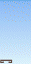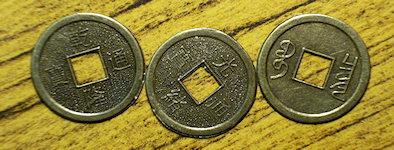# Consulting the Yi Jing 易经I Ching WG (Book of Changes)

There are a variety of methods for casting a Yi Jing (I-Ching), to adapt to modern times where speed is a factor, shortcuts are often used. The oldest and most complex method uses Yarrow sticks which produces different outcome probabilities compared to modern versions.

## Shaking a vase

Sixty four sticks are placed loosely in a vase, each stick is marked with one hexagram. The vase is then tilted at an angle and shaken until one of the sticks slowly works itself loose and falls out of the vase. This method yields one gua (hexagram) each time .

## Tossing coins

It was as long ago as the Tang dynasty that coins started to be used to cast a Yi Jing. Sets of three ‘I Ching’ coins were, and continued to be, minted specifically for this purpose. There are two distinct methods:

### Six coin tosses

Only one coin is needed and all you do to build a hexagram is to toss it six times and record the outcomes. Traditionally heads are counted as yang and tails as yin. The Yi Jing hexagram is built from the bottom up.### Six sets of Three coin tosses

This is a much more authentic method as it produces two hexagrams. The three coins are tossed to give each line of the I Ching hexagram starting, as ever from the bottom. Three heads give changing yang; two heads (one tail) unchanging yin; one head (two tails) unchanging yang and three tails gives changing yin. The probability of a changing yin or yang is 12.5% (1 in 8) and for unchanging 37.5% (3 in 8), so this method does favor the stable forms, but yin and yang are equally likely.Representation for an unchanging or young Yang line. Two heads.Representation for an unchanging or young Yin line. One head.Representation for a changing or old Yang line that is transforming to Yin. Three heads.Representation for a changing or old Yin line that is transforming to Yang. Three tails.1 tail and 2 heads in Yi Jing (I Ching) coins Image by CD343 available under a Creative Commons License . Head-Head-Tail = Unchanging Yin## I Ching with Yarrow sticks

The yarrow stick method is considered the most ancient and authentic way of consulting the Yi Jing, but it can take several minutes to cast a hexagram.

Yarrow sticks are stalks of the herb Achillea sibirica (Siberian Yarrow). It is a close relative of the ornamental plant 'Achillea' grown in gardens. Yarrow was used medicinally to both staunch bleeding and cause nose bleeding giving it a powerful association with life. In Asia a simple method of divination was to insert the foliage in the nose and the answer was determined by whether or not the nose started to bleed. The reason for the Western botanical name ‘Achillea’ was chosen because Achilles used it to staunch the bleeding of his troops according to the Illiad .

50 yarrow sticks are used, the first step is to take out one stick and set it down parallel in front of you, this is the 'observer' and takes no further part in the process. This leaves 49 sticks. The following steps are repeated six times once for each line of the hexagram starting from the bottom towards the top.1. Divide the bunch of sticks into two bundles and take these in either hand. This is where the diviner needs to concentrate on the question to be answered as it is the division of the sticks that determines the outcome.
2. Put the right hand bundle down, and take one stick from this bundle and put it in between the little finger and ring finger of your left hand.
3. Now count out the bundle in your left hand in groups of four onto the table until four or fewer sticks remain. Put these remaining sticks between the ring and middle finger of the left hand.
4. Turn your attention to the right hand bundle you put down, pick it up and now count them out in fours just like the other bundle. Put the remaining sticks (always between one and four) in your left hand and gather them together with the sticks between your fingers into one bundle and place them cross-ways on the single 'observer' stick that you started with.
5. Pick up all the sticks (except those on the observer) and repeat steps 1-4, gathering up the remainders and placing them on the ‘observer’ to make a second pile on the observer stick.
6. One last time, gather up all the sticks and repeat steps 1-4, gathering up the remainders and placing them on the ‘observer’ again. You will now have three little bundles of sticks laid out on the ‘observer’ stick. Count up the number of sticks in each little bundle and note down the total. These three numbers generate the first bottom line of the hexagram.
7. Now you need to repeat all the steps 1-6 five more times building up the hexagram by one line at a time, from the bottom upwards.Accumulation of the remainders 9, 4 and 8 using three divisions which is interpreted as an unchanging Yang line.

### Yarrow stick method probabilities

The mechanism of counting in fours is a little more complex than you might first think. As the remainders can be 1 to 4 you might expect the counts of the groups to be anything from 1+1=2 all the way up to 4+4=8 but this is not the case. In the first round there were 49 sticks, the second and third rounds only used the 'fours' so they are always a multiple of four. To appreciate how it works you need to go through the mathematics. If there are 'n' sticks in the left hand, then the first remainder of the left hand pile can be 1, 2, 3 or 4 sticks but this leaves 49-1-n sticks in the right hand pile. The remainder of the right pile can only be 3, 2, 1 or 4 respectively. So the total for the left and right remainders can only be 4, 4, 4 or 8 respectively. With the extra stick from the right side this requires the total for the first bundle to be only ever 5 or 9. If your total is not 5 or 9 then you have miscounted. In effect you only need to count the sticks in the left hand, those in the right hand just confirm you have counted correctly. You will also note that the probability is not 50:50 as it would be for tossing a coin. This accounts for the different probabilities of outcomes in the yarrow stick method.

In the second and third round of divisions there are a multiple of 4 sticks to start with not the original 49 so the mathematics is different. Although the left hand remainder can again be 1, 2, 3 or 4 sticks for the right hand the remainder can only be 2, 1, 4 or 3 respectively. Adding the two sets of remainders gives 3, 3, 7 or 7. With the extra stick from the right side this requires the total for the second and third bundles to be only ever 4 or 8. If you have a total other than 4 or 8 in total then you have miscounted just as in the first round. However in this case there is an equal probability of getting a total of either 4 or 8, as with tossing a coin.

Now comes the task of converting the counts into one line of the hexagram.
9+8+8 = Changing yin; (chance 0.25x0.5x0.5 = 6.25%)9+8+4 or 9+4+8 or 5+8+8 = yang (chance 6.25+6.25+18.75=31.25%)9+4+4 or 5+4+8 or 5+8+4 = yin (chance 6.25+18.75+18.75=43.75%)5+4+4= Changing yang; (chance 0.75x0.5x0.5 = 18.75%)Note the unequal distribution; changing yin and unchanging yang are less likely so the process favors yin.

To understand the I Ching method with yarrow sticks please try our online Yi Jing consultation that simulates how this method works.